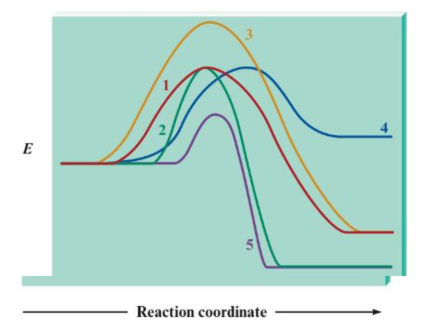# Consider the following potential energy plots: a. Rank the reactions from fastest to slowest and explain your answer. If any reactions have equal rates, explain why. b. Label the reactions as endothermic or exothermic, and support your answer. c. Rank the exothermic reactions from greatest to least change in potential energy. and support your answer.### Chemistry: An Atoms First Approach

2nd Edition
Steven S. Zumdahl + 1 other
Publisher: Cengage Learning
ISBN: 9781305079243

#### Solutions

Chapter
Section### Chemistry: An Atoms First Approach

2nd Edition
Steven S. Zumdahl + 1 other
Publisher: Cengage Learning
ISBN: 9781305079243
Chapter 16, Problem 16Q
Textbook Problem
8 views

## Consider the following potential energy plots:a. Rank the reactions from fastest to slowest and explain your answer. If any reactions have equal rates, explain why.b. Label the reactions as endothermic or exothermic, and support your answer.c. Rank the exothermic reactions from greatest to least change in potential energy. and support your answer.

(a)

Interpretation Introduction

Interpretation: A potential energy plot has been given. Various questions based on this potential energy plot are to be answered.

Concept introduction: A graph that gives information regarding the net energy change during the conversion of the reactants into products is known as the potential energy plot.

### Explanation of Solution

The amount of energy required for a reaction to be initiated is known as activation energy.

Figure 1

The reaction that has a lower value of activation energy corresponding to it will take place faster than the one having a comparatively higher value of activation energy.

The stated potential energy plot is,

(b)

Interpretation Introduction

Interpretation: A potential energy plot has been given. Various questions based on this potential energy plot are to be answered.

Concept introduction: A graph that gives information regarding the net energy change during the conversion of the reactants into products is known as the potential energy plot.

(c)

Interpretation Introduction

Interpretation: A potential energy plot has been given. Various questions based on this potential energy plot are to be answered.

Concept introduction: A graph that gives information regarding the net energy change during the conversion of the reactants into products is known as the potential energy plot.

### Still sussing out bartleby?

Check out a sample textbook solution.

See a sample solution

#### The Solution to Your Study Problems

Bartleby provides explanations to thousands of textbook problems written by our experts, many with advanced degrees!

Get Started

Find more solutions based on key concepts
Vitamin A supplements can help treat acne. T F

Nutrition: Concepts and Controversies - Standalone book (MindTap Course List)

48. Name each alkyne:

Chemistry In Focus

How many moles are contained in a 54.0g sample of Al? a. 1.0 b. 2.0 c. 0.5 d. 4.0

Chemistry for Today: General, Organic, and Biochemistry

In the following diagram, designate each daughter cell as diploid (2n) or haploid (n).

Human Heredity: Principles and Issues (MindTap Course List)

How can the Doppler effect explain shifts in both light and sound?

Horizons: Exploring the Universe (MindTap Course List)

What is triacylglycerol mobilization?

Organic And Biological Chemistry

A rectangular coil with resistance R has N turns, each of length and width as shown in Figure P31.36. The coi...

Physics for Scientists and Engineers, Technology Update (No access codes included)

Whats a buffer? Why is seawaters buffering capacity important to marine life?

Oceanography: An Invitation To Marine Science, Loose-leaf Versin